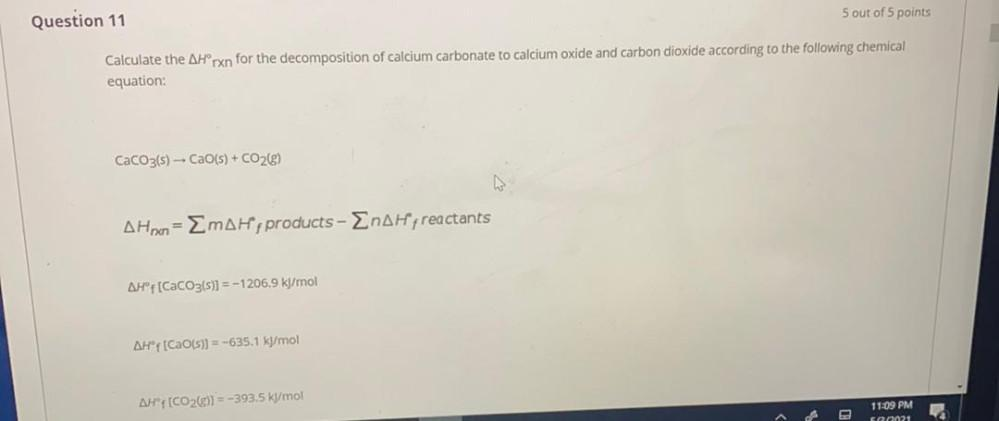Question:

# Calculate the △H°rxn for the decomposition of calcium

Last updated: 7/28/2022Calculate the △H°rxn for the decomposition of calcium carbonate to calcium oxide and carbon dioxide according to the following chemical equation: CaCO₃(s) → CaO(s) + CO₂(g) △Hrxn= Σm△H⁰ f products - Σn△H⁰ f reactants △H° f (CaCO₃(s)] = -1206.9 kJ/mol △H⁰ f [CaOS)] = -635.1 ks/mol △H⁰ f (CO₂) = -393.5 kJ/mol